## Example Questions

### Example Question #1 : How To Find Value With A Number Line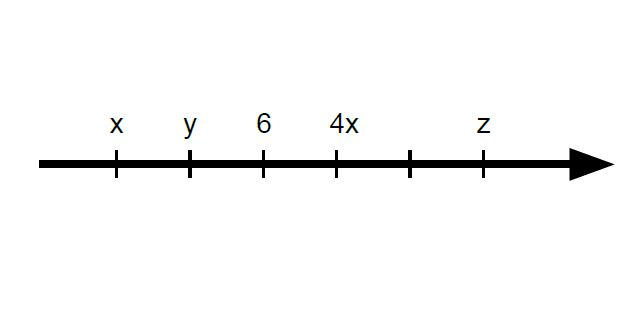If the tick marks are equally spaced on the number line above, what is the average (arithmetic mean) of x, y, and z?

5

7

6

4

8

6

Explanation:

First, we must find out by how much they are spaced by. It cannot be 1, since 4(4) = 16, which is too great of a step in the positive direction and exceeds the equal-spacing limit. 2 works perfectly, however, as 4(2) equals 8 and fits in line with the equal spacing.

Next, we can find the values of x and y since we are given a value of 6 for the third tick mark. As such, x (6 – 4) and y (6 – 2) are 2 and 4, respectively.

Finally, z is 4 steps away from y, and since each step has a value of 2, 2(4) = 8, plus the value that y is already at, 8 + 4 = 12 (or can simply count).

Finding the average of all 3 values, we get (2 + 4 + 12)/3 = 18/3 = 6.

### Example Question #2 : How To Find Value With A Number Line

How many numbers 1 to 250 inclusive are cubes of integers?Explanation:

The cubes of integers from 1 to 250 are 1, 8, 27,64,125,216.

### Example Question #3 : How To Find Value With A Number Line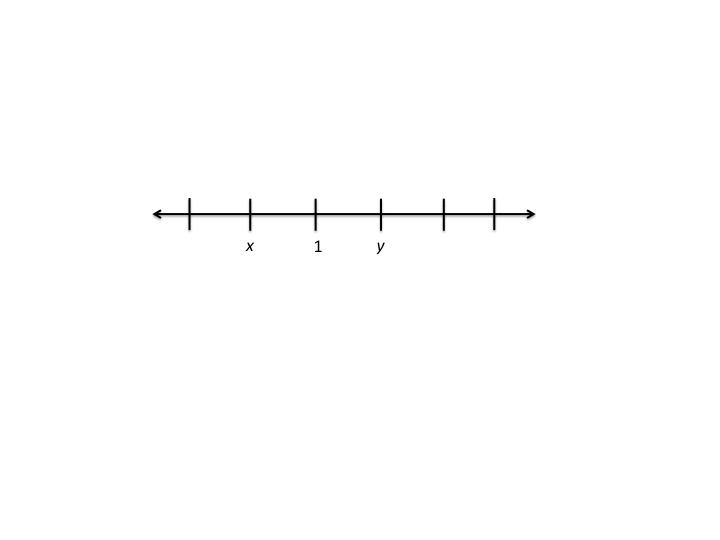On the number line above, the tick marks are equally spaced. Which of the following expressesin terms of?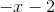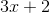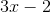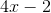Ifis betweenandon the number line, thenand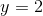.
Somust be correct because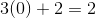.